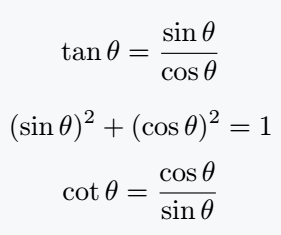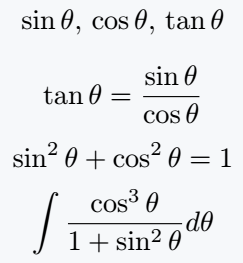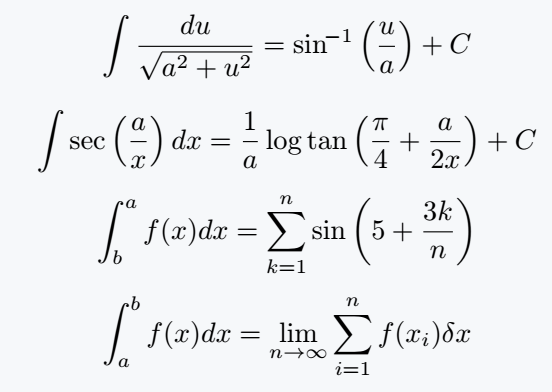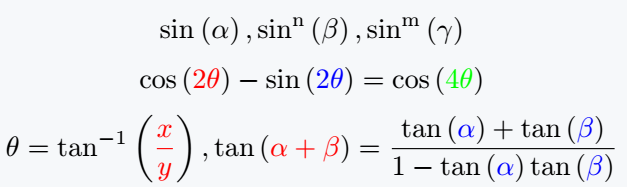# How to use \newcommand in LaTeX?

\newcommand is a command, meaning of work is hidden in its name. So, a \newcommand is a user-defined command that can be used to create new commands with custom functionality.

These commands can be used to simplify repetitive tasks, such as creating consistent formatting or inserting frequently used text.

This tutorial provides a to z information about \newcommand that will expand your knowledge even a little.

## Command structure and syntax

Before seeing the practical use of this command, the syntax and structure must be understood.

\newcommand[cmd][exp]
\newcommand[cmd][number_of_arg][exp]
\newcommand[cmd][number_of_arg][opt_arg][exp]

cmd : will define the name of the custom macro.
arg : will define how many arguments you pass to custom command.
exp : will represent custom macro’s functional work.
opt_arg : will define optional arguments.

Most important thing is that custom command cannot have same name as the existing command.

Following are four points discussed based on Arguments and Optional Arguments which will give you a clear concept.

## Pass no arguments

In this case I will not pass any arguments, just define command name and functional work with it.

\documentclass{article}
\newcommand{\Sin}{\mathrm{sin}\,\theta}
\newcommand{\Cos}{\mathrm{cos}\,\theta}
\newcommand{\Tan}{\mathrm{tan}\,\theta}
\begin{document}
$\Tan = \frac{\Sin}{\Cos}$
$(\Sin)^2 + (\Cos)^2 =1$
$\cot\theta = \frac{\Cos}{\Sin}$
\end{document}

Output :But, even if command is defined with same name in code above, the two commands will be treated as separate commands in LaTeX.

Because LaTeX is a case sensitive markup language.

## Pass one argument

Second, I would create a new macro but pass it an argument.

\newcommand{macro name}{exp}


where macro name is the name you want to give to your custom command,  represents that it takes one argument, and exp will replace be your custom command when it is used in the document.

\documentclass{article}
\newcommand{\trig}{\mathrm{#1}\,\theta}
\begin{document}
$\trig{sin},\,\trig{cos},\,\trig{tan}$
$\trig{tan} = \frac{\trig{sin}}{\trig{cos}}$
$\trig{sin^2} + \trig{cos^2} =1$
$\int \frac{\trig{cos^3}}{1+\trig{sin^2}}d\theta$
\end{document}

Output :## Pass more than one arguments

Third, you can add more functionality by passing more than one argument when creating a custom macro.

\documentclass{article}
\newcommand{\trig}{\mathrm{#1}\left(#2\right)}
\newcommand{\Int}{\int_{#2}^{#1}}
$\int\frac{du}{\sqrt{a^2 + u^2}}=\trig{sin^{\!-1}}{\frac{u}{a}} + C$
$\int\trig{sec}{\frac{a}{x}}dx = \frac{1}{a} \log\trig{tan}{\frac{\pi}{4}+ \frac{a}{2x}} + C$
$\Int{a}{b}f(x)dx = \sum_{k=1}^n \trig{sin}{5+\frac{3k}{n}}$
$\Int{b}{a}f(x)dx = \lim_{n \to \infty} \sum_{i=1}^{n}f(x_i)\delta x$
\end{document}

Output :## Pass optional argument

We’ve seen many of LaTeX’s built-in commands. Where optional arguments are used. Which makes the functionality of the command more beautiful.

When you define a command instead of built-in, how to add optional arguments, the syntax is given below.

\newcommand[cmd][arg][number_of_arg][exp]
\documentclass{article}
\usepackage{xcolor}
\newcommand{\trig}[]{\mathrm{#2^{#1}}\left(#3\right)}
\newcommand{\trigx}[]{\mathrm{#2}\left({\color{#1}#3}\right)}
\begin{document}
$\trig{sin}{\alpha}, \trig[n]{sin}{\beta},\trig[m]{sin}{\gamma}$
$\trigx[red]{cos}{2\theta}-\trigx[blue]{sin}{2\theta}=\trigx[green]{cos}{4\theta}$
$\theta=\trigx[red]{tan^{-1}}{\frac{x}{y}},\trigx[red]{tan}{\alpha+\beta}=\frac{\trigx[blue]{tan}{\alpha}+\trigx[blue]{tan}{\beta}}{1-\trigx[blue]{tan}{\alpha}\trigx[blue]{tan}{\beta}}$
\end{document}

Output :The tutorial ends here. But, there is one remaining topic related to this tutorial that is “\renewcomamnd” which will be discussed in another tutorial.#### Md Jidan Mondal

LaTeX expert with over 10 years of experience in document preparation and typesetting. Specializes in creating professional documents, reports, and presentations using LaTeX.